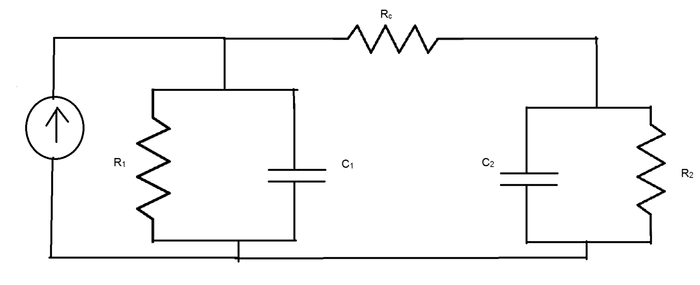# Coupled RC circuit

• Engineering

## Homework Statement

Hello I want to solve the following circuit, I want to know how the voltage on R1 and R2 develop with time,
It start at t=0 when there is no voltage in the circuit ( all V=0). Its a symbolic question, I need to show the equation.
V1(t) = ? ,
V2(t) = ?
The current is a DC

## Homework Equations## The Attempt at a Solution

Well, noting much.. I just wrote it in a better way:
http://i62.tinypic.com/5w9gxz.png

VR1 = VC1
VR1 + VRc + VC2 = 0
VR2 = VC2
IR1 + IC1 + VRC = Iinj
IRc = IR2 + VC2
$$V_1 = V_{C1} = V_{R1} \\ V_2 = V_{R2} = V_{C2}$$

$$\frac{V_1}{R_1} + \frac{dV_1}{dt}*C_1 + \frac{V_{Rc}}{R_c} = I_{inj} \\ \frac{V_{Rc}}{R_c} = \frac{V_2}{R_2} + C_2*\frac{dV_2}{dt}$$

-->

$$\frac{dV_1}{dt}*C_1 = I_{inj} -\frac{V_{Rc}}{R_c} - \frac{V_1}{R_1} \\ \frac{dV_2}{dt}*C_2 = \frac{V_2}{R_2} -\frac{V_{Rc}}{R_c}$$

Last edited:

donpacino
Gold Member
Do you know how to do nodal analysis?

evaluate the circuit in the frequency domain using nodal analysis.

In case you don't know nodal analysis, simply write a KCL at node V1 and V2 and solve.

gneill
Mentor
You haven't defined the question sufficiently. Do you have component values or is this strictly a symbolic problem? What are the properties of the current source? Is it a DC source or an AC source? Any initial conditions?

Hi @gneill and @donpacino thank you for the replay, I added some info about the question.
I also tried to make some progress.

donpacino
Gold Member
I would start by defining the node in the upper left as V1, the node in the upper right as V2, and the bottom node as ground. so VRc=V1-V2, V1=VR1=VC1, etc
Do a laplace transform (will allow you to solve the system with algebra) to get to the frequency domain.

write two kcls at the upper left and right nodes using V1,V2,Iinj,R1,R2,Rc,C1,C2
then do some algebra and do an inverse laplace transform to get to your time domain solution.

alternatively you can solve the second order differential equation.

just a note: I am operating under the assumption that at t=0 the current source changes values. If it does not then you can disregard the capacitors.

thank you donpacino but I do not know how to do laplace transform...

donpacino
Gold Member
ok, then do what i told you, but solve the differential equation in the time domain. you will still do a KCL, but in the time domain similar to what you did above